Year 1 - Recognise and describe one-half as one of two equal parts of a whole.
Australian Curriculum Yr 1 Achievement Standard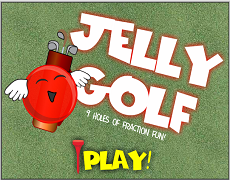### Jelly GolfTablet ✅

Swing the golf club as far as the fraction.

Back to TopYear 2 - Divide collections and shapes into halves, quarters and eighths. Students recognise and interpret common uses of halves, quarters and eighths of shapes and collections. Australian Curriculum Yr 2 Achievement Standard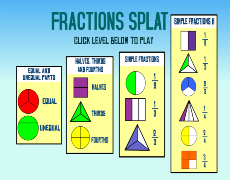### Fraction Splat NEW!Tablet ✅

Splat the matching fractions. 4 levels.### Jelly GolfTablet ✅

Swing the golf club as far as the fraction.

Back to TopYear 3 - Model and represent unit fractions including 1/2, 1/4, 1/3, 1/5 and their multiples to a complete whole.
Australian Curriculum Yr 3 Achievement Standard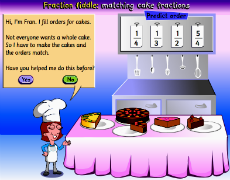### Cake FractionsTablet ✅

Equivalent fractions including mixed numerals.### Fraction Splat NEW!Tablet ✅

Splat the matching fractions. 4 levels.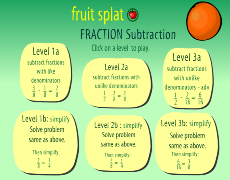### Fraction Splat - Subtraction NEW!Tablet ✅

Match the correct subtracted fraction to the answer. 6 levels.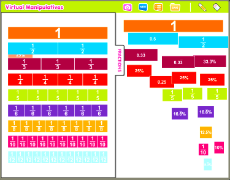### Fraction, Percent and Decimal tiles

How to make a whole.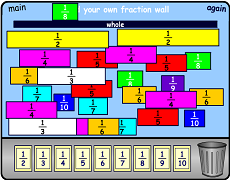### FractionsTablet ✅

Showing fractions, decimals and how they are related.### Jelly GolfTablet ✅

Swing the golf club as far as the fraction.

Back to TopYear 4 - Recognise common equivalent fractions in familiar contexts and make connections between fraction and decimal notations up to two decimal places. Locate familiar fractions on a number line.
Australian Curriculum Yr 4 Achievement Standard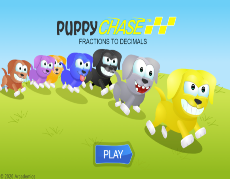### Convert Puppy FactionsTablet ✅

Power up your puppy by changing fractions to decimals.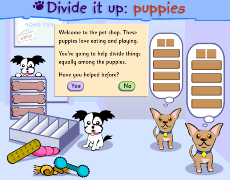### Divide it Up: Puppies NEW!Tablet ✅

Divide toys up for the puppies using a dividing tool.### Fraction Splat NEW!Tablet ✅

Splat the matching fractions. 4 levels.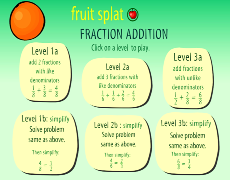### Fraction Splat - Addition NEW!Tablet ✅

Match the correct added fraction to the answer. 6 levels.### Fraction Splat - Subtraction NEW!Tablet ✅

Match the correct subtracted fraction to the answer. 6 levels.### Fraction, Percent and Decimal tiles

How to make a whole.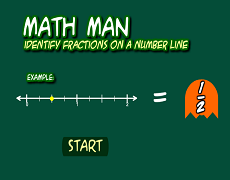### Fractions on a Number Line

Gobble ghosts on the fraction number line.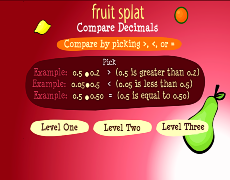### Fruit Splat - Compare decimals NEW!Tablet ✅

Compare decimals by choosing , or =. 3 levels.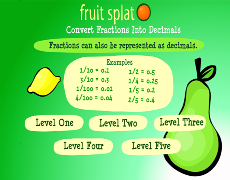### Fruit Splat - Fractions to Decimals NEW!Tablet ✅

Match the fraction to the decimal. 6 levels.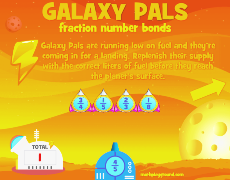### Galaxy Fraction PalsTablet ✅

Shoot the ship that has the fraction to make the whole### Jelly GolfTablet ✅

Swing the golf club as far as the fraction.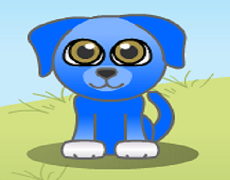### Puppy Chase DecimalsTablet ✅

Match the fraction to the decimal.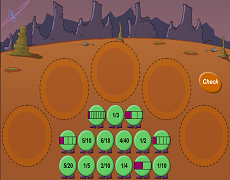### Space Triplets 1Tablet ✅

Make groups of equivalent fractions.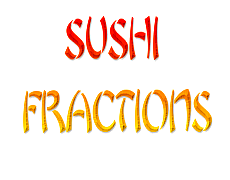### Sushi FractionsTablet ✅

Drag fraction ingredients to make sushi.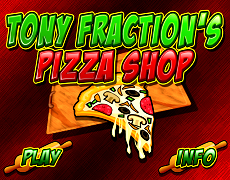### Tony's Fraction Pizza ShopTablet ✅

Read the order and make the pizza!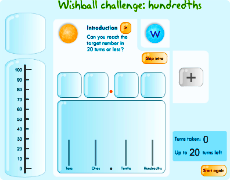### Wishball: 100thsTablet ✅

Reach the target in as few turns as you can.

Back to TopOrder decimals and unit fractions and locate them on number lines. Students add and subtract fractions with the same denominator. Students continue patterns by adding and subtracting fractions and decimals.
Year 5 Australian Curriculum Achievement Standard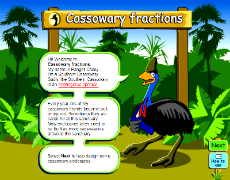### Cassowary FractionsTablet ✅

Build enclosures for sick and injured birds.### Convert Puppy FactionsTablet ✅

Power up your puppy by changing fractions to decimals.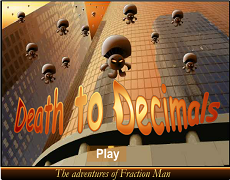### Death to DecimalsTablet ✅

Match decimals to fractions and shoot calculators!### Divide it Up: Puppies NEW!Tablet ✅

Divide toys up for the puppies using a dividing tool.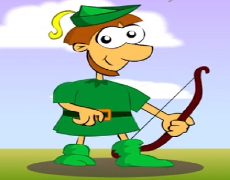### Fraction Fiddle NEW!Tablet ✅

Build two fractions that equal one whole.### Fraction Splat NEW!Tablet ✅

Splat the matching fractions. 4 levels.### Fraction Splat - Addition NEW!Tablet ✅

Match the correct added fraction to the answer. 6 levels.### Fraction Splat - Subtraction NEW!Tablet ✅

Match the correct subtracted fraction to the answer. 6 levels.### Fraction, Percent and Decimal tiles

How to make a whole.### FractionsTablet ✅

Showing fractions, decimals and how they are related.### Fruit Splat - Compare decimals NEW!Tablet ✅

Compare decimals by choosing , or =. 3 levels.### Fruit Splat - Fractions to Decimals NEW!Tablet ✅

Match the fraction to the decimal. 6 levels.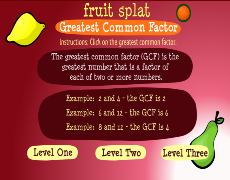### Fruit Splat - Greatest Common Denominator NEW!Tablet ✅

Splat the greatest common denominator### Galaxy Fraction PalsTablet ✅

Shoot the ship that has the fraction to make the whole### Jelly GolfTablet ✅

Swing the golf club as far as the fraction.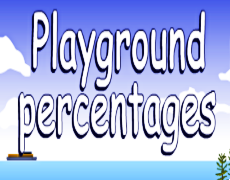### Playground Percentages NEW!Tablet ✅

Calculate the percentages/fractions of a site to build your own playground.### Space Triplets 1Tablet ✅

Make groups of equivalent fractions.### Wishball: 100thsTablet ✅

Reach the target in as few turns as you can.

Back to TopYear 6 - Connect fractions, decimals and percentages as different representations of the same number. Students solve problems involving the addition and subtraction of related fractions. They calculate a simple fraction of a quantity. Students add, subtract and multiply decimals and divide decimals where the result is rational. Students calculate common percentage discounts on sale items. Australian Curriculum Yr 6 Achievement Standard### Convert Puppy FactionsTablet ✅

Power up your puppy by changing fractions to decimals.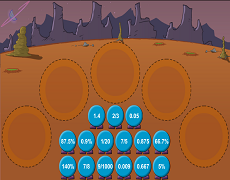### Decent - fractions, decimals & %Tablet ✅

Match values at the Space Games.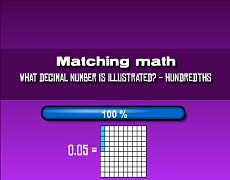### Decimals 100th Match

Which picture represents the decimal?### Divide it Up: Puppies NEW!Tablet ✅

Divide toys up for the puppies using a dividing tool.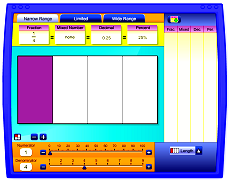### Explore Fractions, Decimals & %Tablet ✅

Explore fractions, mixed numbers, decimals & %.### Fraction Fiddle NEW!Tablet ✅

Build two fractions that equal one whole.### Fraction Splat - Addition NEW!Tablet ✅

Match the correct added fraction to the answer. 6 levels.### Fraction Splat - Subtraction NEW!Tablet ✅

Match the correct subtracted fraction to the answer. 6 levels.### Fraction, Percent and Decimal tiles

How to make a whole.### Fruit Splat - Compare decimals NEW!Tablet ✅

Compare decimals by choosing , or =. 3 levels.### Fruit Splat - Fractions to Decimals NEW!Tablet ✅

Match the fraction to the decimal. 6 levels.### Fruit Splat - Greatest Common Denominator NEW!Tablet ✅

Splat the greatest common denominator### Galaxy Fraction PalsTablet ✅

Shoot the ship that has the fraction to make the whole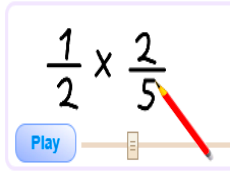### Multiplying FractionsTablet ✅

A step by step guide to mutiplying fractions.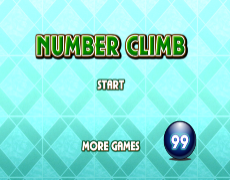### Number ClimbTablet ✅

Shoot the numbers in the correct order.### Playground Percentages NEW!Tablet ✅

Calculate the percentages/fractions of a site to build your own playground.### Space Triplets 1Tablet ✅

Make groups of equivalent fractions.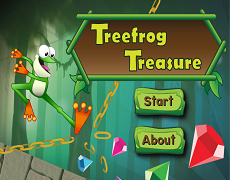### Treefrog Treasure

Collect jewels by matching decimal, fraction & percentage.### Wishball: 100thsTablet ✅

Reach the target in as few turns as you can.

Back to TopYear 7 - Solve problems involving percentages and all four operations with fractions and decimals. Students use fractions, decimals and percentages, and their equivalences. They express one quantity as a fraction or percentage of another.
Australian Curriculum Yr 7 Achievement Standard### Convert Puppy FactionsTablet ✅

Power up your puppy by changing fractions to decimals.### Divide it Up: Puppies NEW!Tablet ✅

Divide toys up for the puppies using a dividing tool.### Fraction Fiddle NEW!Tablet ✅

Build two fractions that equal one whole.### Fraction Splat - Addition NEW!Tablet ✅

Match the correct added fraction to the answer. 6 levels.### Fraction Splat - Subtraction NEW!Tablet ✅

Match the correct subtracted fraction to the answer. 6 levels.### Fraction, Percent and Decimal tiles

How to make a whole.### Fruit Splat - Compare decimals NEW!Tablet ✅

Compare decimals by choosing , or =. 3 levels.### Fruit Splat - Fractions to Decimals NEW!Tablet ✅

Match the fraction to the decimal. 6 levels.### Fruit Splat - Greatest Common Denominator NEW!Tablet ✅

Splat the greatest common denominator### Galaxy Fraction PalsTablet ✅

Shoot the ship that has the fraction to make the whole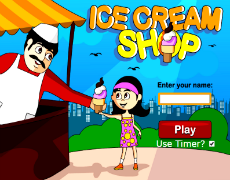### Ice Cream ShopTablet ✅

Can you fill customer ice cream orders?### Multiplying FractionsTablet ✅

A step by step guide to mutiplying fractions.### Number ClimbTablet ✅

Shoot the numbers in the correct order.### Playground Percentages NEW!Tablet ✅

Calculate the percentages/fractions of a site to build your own playground.### Space Triplets 1Tablet ✅

Make groups of equivalent fractions.### Wishball: 100thsTablet ✅

Reach the target in as few turns as you can.